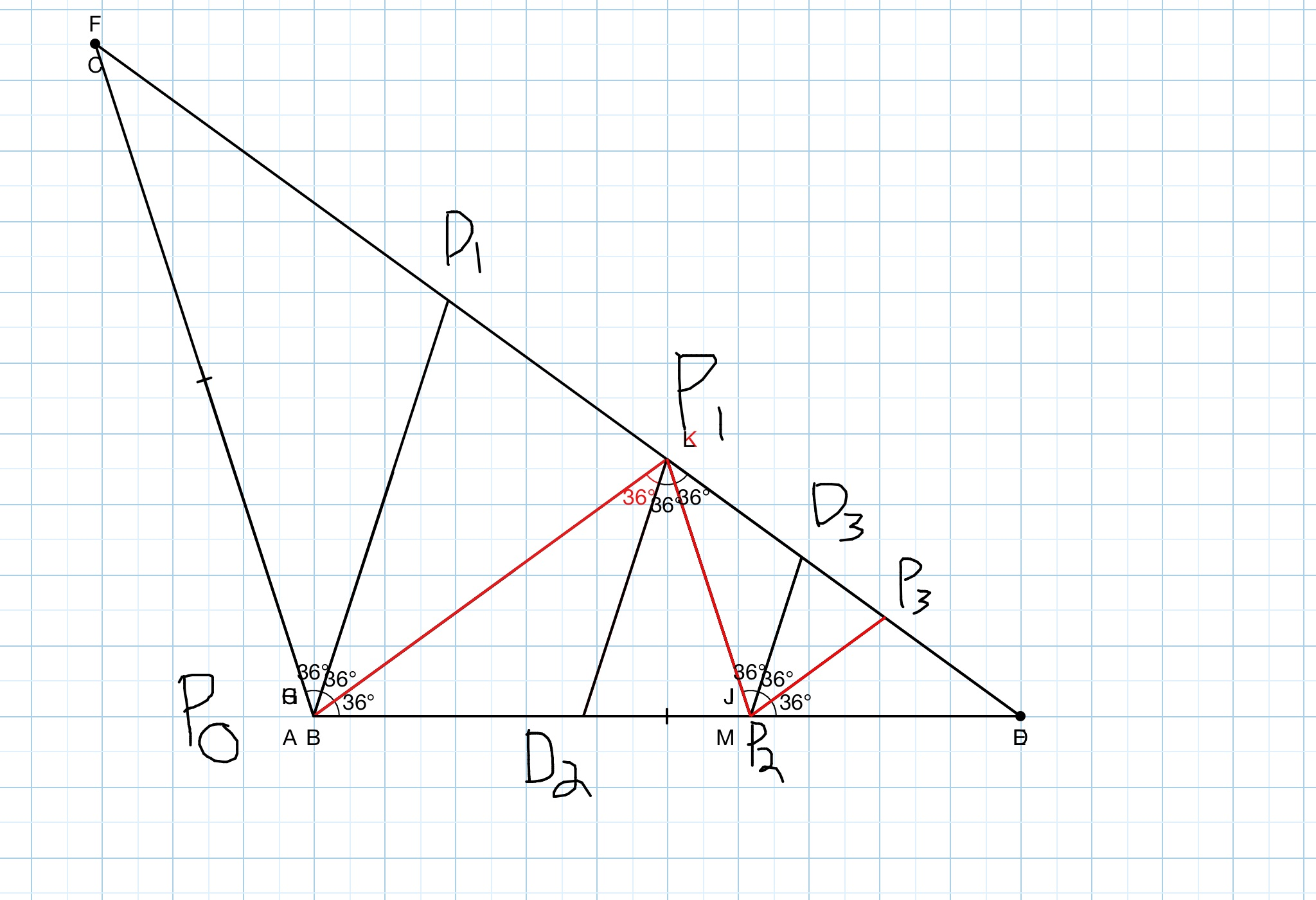# Angle trisection: A Beautiful problem

Geometry Level 5Note: I give two explanations for this problem (both are the same thing) to make the problem more understandable.

Let triangle $\triangle ABP_0$ be an obtuse isosceles triangle with $\angle B=36^{\circ}$, $\angle P_0=108^{\circ}$, and segment $\overline{AB}=2015$.

Here is a general description of the pattern.

Angle $\angle BP_0A$ is trisected by segments $\overline{P_0 P_1}$ and $\overline{P_0 D_1}$ where $P_1$ and $D_1$ are on side $\overline{AB}$ and $\overline{BP_1}<\overline{BD_1}$

Next, angle $P_0P_1B$ is trisected by segments $\overline{P_1P_2}$ and $\overline{P_1D_2}$ where $P_1$ and $D_1$ are on side $\overline{P_0B}$ and $\overline{P_2B}<\overline{D_2B}$.

Here is an explicit description

Now, given the pattern above, let the point $P_n$ and $D_n$ be the points resulting from the trisection of $\angle P_{n-2}P_{n-1}B$ with $\overline{BP_n}<\overline{BD_n}$.

If $\dfrac{\overline{P_9P_{10}}}{\overline{P_6P_7}}$ can be expressed as $\left(\dfrac{-a+\sqrt{b}}{c}\right)^k$ where $b$ is square free and $a,c$ are co-prime positive integers and $k$ is an integer, what is the value of $abck$?

This is part of the set Trevor's Ten

Details and Assumptions

• $\cos{36}=\dfrac{1+\sqrt{5}}{4}$. (And I know what you're thinking, the answer is not 20. Lol, if only it were that simple)

• Hint: make an equation for $\overline{P_{n-1}P_{n}}$. Don't work with numbers.

###### By the way, this problem is VERY beautiful and is without a doubt my new favorite problem that I've made.
×

Problem Loading...

Note Loading...

Set Loading...